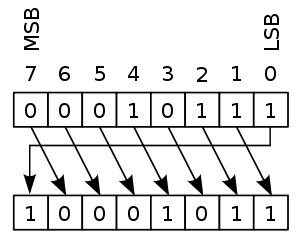# Circular shift

In combinatorial mathematics, a circular shift is the operation of rearranging the entries in a tuple, either by moving the final entry to the first position, while shifting all other entries to the next position, or by performing the inverse operation. A circular shift is a special kind of cyclic permutation, which in turn is a special kind of permutation. Formally, a circular shift is a permutation σ of the n entries in the tuple such that either

$\sigma (i)\equiv (i+1)$modulo n, for all entries i = 1, ..., n

or

$\sigma (i)\equiv (i-1)$modulo n, for all entries i = 1, ..., n.

The result of repeatedly applying circular shifts to a given tuple are also called the circular shifts of the tuple.

For example, repeatedly applying circular shifts to the four-tuple (a, b, c, d) successively gives

• (d, a, b, c),
• (c, d, a, b),
• (b, c, d, a),
• (a, b, c, d) (the original four-tuple),

and then the sequence repeats; this four-tuple therefore has four distinct circular shifts. However, not all n-tuples have n distinct circular shifts. For instance, the 4-tuple (a, b, a, b) only has 2 distinct circular shifts. In general the number of circular shifts of an n-tuple could be any divisor of n, depending on the entries of the tuple.

In computer programming, a bitwise rotation, also known as a circular shift, is a bitwise operation that shifts all bits of its operand. Unlike an arithmetic shift, a circular shift does not preserve a number's sign bit or distinguish a floating-point number's exponent from its significand. Unlike a logical shift, the vacant bit positions are not filled in with zeros but are filled in with the bits that are shifted out of the sequence.

## Implementing circular shifts

Circular shifts are used often in cryptography in order to permute bit sequences. Unfortunately, many programming languages, including C, do not have operators or standard functions for circular shifting, even though virtually all processors have bitwise operation instructions for it (e.g. Intel x86 has ROL and ROR). However, some compilers may provide access to the processor instructions by means of intrinsic functions. In addition, some constructs in standard ANSI C code may be optimized by a compiler to the "rotate" assembly language instruction on CPUs that have such an instruction. Most C compilers recognize the following idiom, and compile it to a single 32-bit rotate instruction.

/*
* Shift operations in C are only defined for shift values which are
* not negative and smaller than sizeof(value) * CHAR_BIT.
* The mask, used with bitwise-and (&), prevents undefined behaviour
* when the shift count is 0 or >= the width of unsigned int.
*/

#include <stdint.h>  // for uint32_t, to get 32-bit-wide rotates, regardless of the size of int.
#include <limits.h>  // for CHAR_BIT

uint32_t rotl32 (uint32_t value, unsigned int count) {
const unsigned int mask = CHAR_BIT * sizeof(value) - 1;
return (value << count) | (value >> (-count & mask));
}

uint32_t rotr32 (uint32_t value, unsigned int count) {
const unsigned int mask = CHAR_BIT * sizeof(value) - 1;
return (value >> count) | (value << (-count & mask));
}


This safe and compiler-friendly implementation was developed by John Regehr, and further polished by Peter Cordes.

A simpler version is often seen when the count is limited to the range of 1 to 31 bits:

uint32_t rotl32 (uint32_t value, unsigned int count) {
return value << count | value >> (32 - count);
}


This version is dangerous because if the count is 0 or 32, it asks for a 32-bit shift, which is undefined behaviour in the C language standard. However, it tends to work anyway, because most microprocessors implement value >> 32 as either a 32-bit shift (producing 0) or a 0-bit shift (producing the original value), and either one produces the correct result in this application.

## Example

If the bit sequence 0001 0111 were subjected to a circular shift of one bit position... (see images below)

 to the left would yield: 0010 1110 to the right would yield: 1000 1011.Right circular shift.

If the bit sequence 1001 0110 were subjected to the following operations:

 left circular shift by 1 position: 0010 1101 left circular shift by 2 positions: 0101 1010 left circular shift by 3 positions: 1011 0100 left circular shift by 4 positions: 0110 1001 left circular shift by 5 positions: 1101 0010 left circular shift by 6 positions: 1010 0101 left circular shift by 7 positions: 0100 1011 left circular shift by 8 positions: 1001 0110
 right circular shift by 1 position: 0100 1011 right circular shift by 2 positions: 1010 0101 right circular shift by 3 positions: 1101 0010 right circular shift by 4 positions: 0110 1001 right circular shift by 5 positions: 1011 0100 right circular shift by 6 positions: 0101 1010 right circular shift by 7 positions: 0010 1101 right circular shift by 8 positions: 1001 0110

## Applications

Cyclic codes are a kind of block code with the property that the circular shift of a codeword will always yield another codeword. This motivates the following general definition: For a string s over an alphabet Σ, let shift(s) denote the set of circular shifts of s, and for a set L of strings, let shift(L) denote the set of all circular shifts of strings in L. If L is a cyclic code, then shift(L) ⊆ L; this is a necessary condition for L being a cyclic language. The operation shift(L) has been studied in formal language theory. For instance, if L is a context-free language, then shift(L) is again context-free. Also, if L is described by a regular expression of length n, there is a regular expression of length O(n3) describing shift(L).